## Cyclic Pentagon

A cyclic pentagon is a not necessarily regular Pentagon on whose Vertices a Circle may be Circumscribed. Let(1)

where the Sum runs over all distinct Permutations of the Squares of the 5 side lengths, so(2)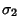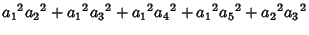(3)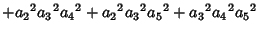(4)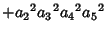(5)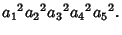(6)

Then define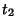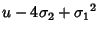(7)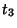(8)(9)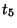(10)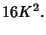(11)

The Area of the pentagon then satisfies(12)

a seventh order Polynomial in. This is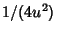times the Discriminant of the Cubic Equation(13)

Robbins, D. P. Areas of Polygons Inscribed in a Circle.'' Discr. Comput. Geom. 12, 223-236, 1994.
Robbins, D. P. Areas of Polygons Inscribed in a Circle.'' Amer. Math. Monthly 102, 523-530, 1995.